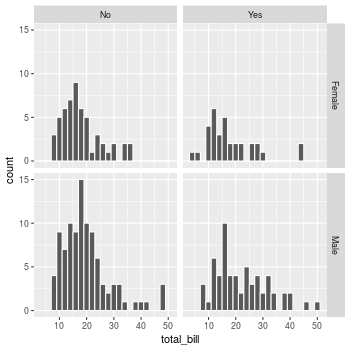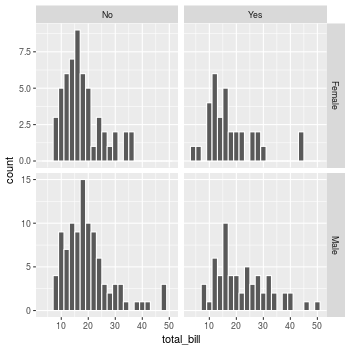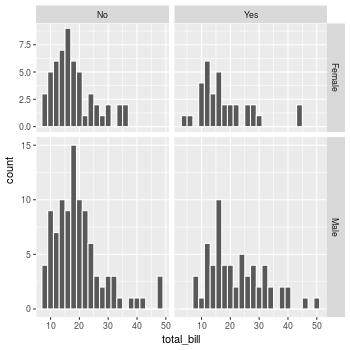## Problem

You want to do split up your data by one or more variables and plot the subsets of data together.

## Solution

### Sample data

We will use the `tips` dataset from the `reshape2` package.

``````library(reshape2)
# Look at first few rows
#>   total_bill  tip    sex smoker day   time size
#> 1      16.99 1.01 Female     No Sun Dinner    2
#> 2      10.34 1.66   Male     No Sun Dinner    3
#> 3      21.01 3.50   Male     No Sun Dinner    3
#> 4      23.68 3.31   Male     No Sun Dinner    2
#> 5      24.59 3.61 Female     No Sun Dinner    4
#> 6      25.29 4.71   Male     No Sun Dinner    4
``````

This is a scatterplot of the tip percentage by total bill size.

``````library(ggplot2)
sp <- ggplot(tips, aes(x=total_bill, y=tip/total_bill)) + geom_point(shape=1)
sp
``````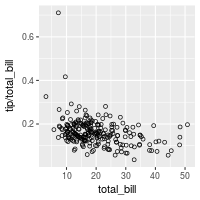### facet_grid

The data can be split up by one or two variables that vary on the horizontal and/or vertical direction.

This is done by giving a formula to `facet_grid()`, of the form `vertical ~ horizontal`.

``````# Divide by levels of "sex", in the vertical direction
sp + facet_grid(sex ~ .)
``````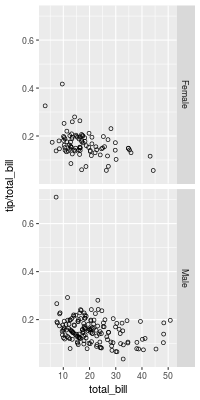``````# Divide by levels of "sex", in the horizontal direction
sp + facet_grid(. ~ sex)
``````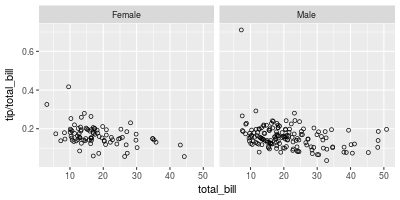``````# Divide with "sex" vertical, "day" horizontal
sp + facet_grid(sex ~ day)
``````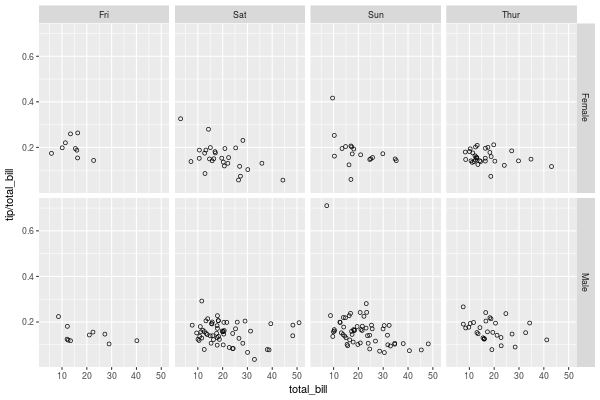### facet_wrap

Instead of faceting with a variable in the horizontal or vertical direction, facets can be placed next to each other, wrapping with a certain number of columns or rows. The label for each plot will be at the top of the plot.

``````# Divide by day, going horizontally and wrapping with 2 columns
sp + facet_wrap( ~ day, ncol=2)
``````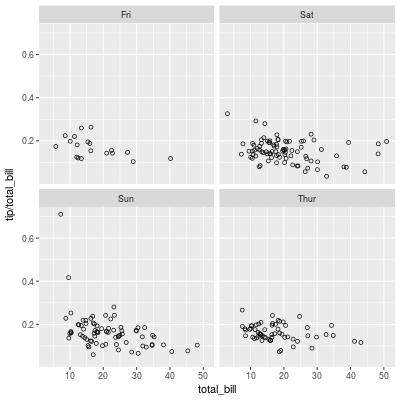### Modifying facet label appearance

``````sp + facet_grid(sex ~ day) +
theme(strip.text.x = element_text(size=8, angle=75),
strip.text.y = element_text(size=12, face="bold"),
strip.background = element_rect(colour="red", fill="#CCCCFF"))
``````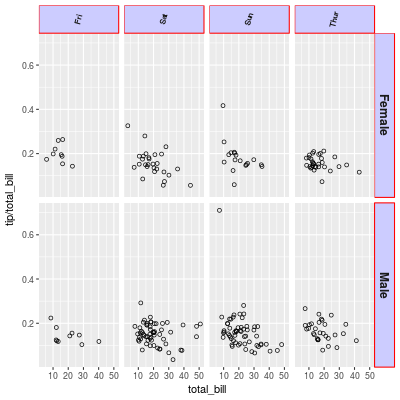### Modifying facet label text

There are a few different ways of modifying facet labels. The simplest way is to provide a named vector that maps original names to new names. To map the levels of `sex` from Female==>Women, and Male==>Men:

``````labels <- c(Female = "Women", Male = "Men")
sp + facet_grid(. ~ sex, labeller=labeller(sex = labels))
``````

Another way is to modify the data frame so that the data contains the desired labels:

``````tips2 <- tips
levels(tips2\$sex)[levels(tips2\$sex)=="Female"] <- "Women"
levels(tips2\$sex)[levels(tips2\$sex)=="Male"]   <- "Men"
#>   total_bill  tip   sex smoker day   time size
#> 1      16.99 1.01 Women     No Sun Dinner    2
#> 2      10.34 1.66   Men     No Sun Dinner    3
#> 3      21.01 3.50   Men     No Sun Dinner    3

# Both of these will give the same output:
sp2 <- ggplot(tips2, aes(x=total_bill, y=tip/total_bill)) + geom_point(shape=1)
sp2 + facet_grid(. ~ sex)
``````

Both of these will give the same result: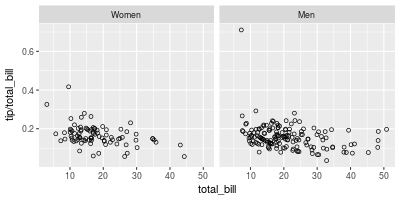`labeller()` can use any function that takes a character vector as input and returns a character vector as output. For example, the `capitalize` function from the Hmisc package will capitalize the first letters of strings. We can also define our own custom functions, like this one, which reverses strings:

``````# Reverse each strings in a character vector
reverse <- function(strings) {
strings <- strsplit(strings, "")
vapply(strings, function(x) {
paste(rev(x), collapse = "")
}, FUN.VALUE = character(1))
}

sp + facet_grid(. ~ sex, labeller=labeller(sex = reverse))
``````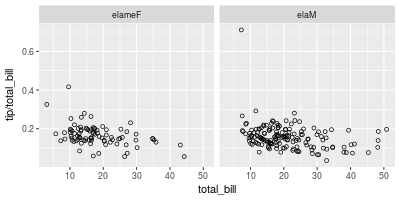### Free scales

Normally, the axis scales on each graph are fixed, which means that they have the same size and range. They can be made independent, by setting `scales` to `free`, `free_x`, or `free_y`.

``````# A histogram of bill sizes
hp <- ggplot(tips, aes(x=total_bill)) + geom_histogram(binwidth=2,colour="white")

# Histogram of total_bill, divided by sex and smoker
hp + facet_grid(sex ~ smoker)

# Same as above, with scales="free_y"
hp + facet_grid(sex ~ smoker, scales="free_y")

# With panels that have the same scaling, but different range (and therefore different physical sizes)
hp + facet_grid(sex ~ smoker, scales="free", space="free")
``````